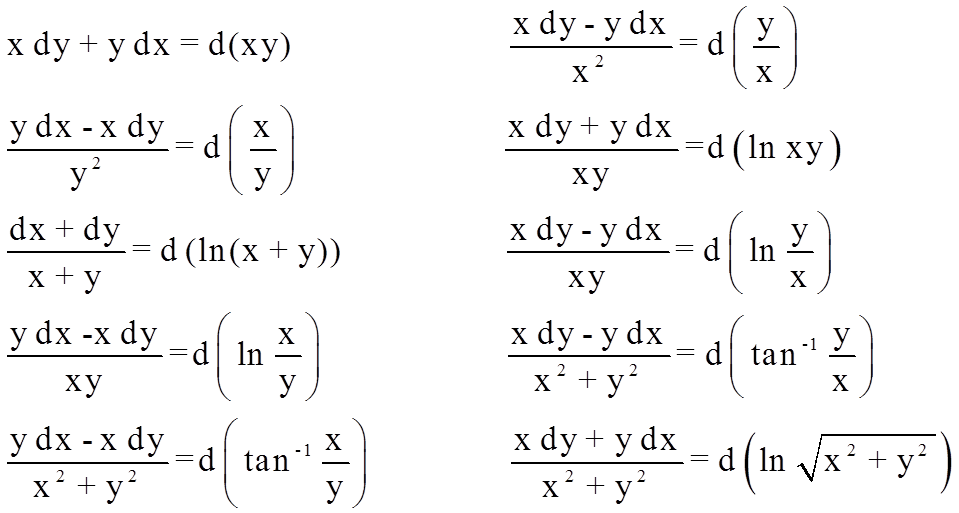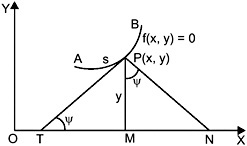×#### Thank you for registering.

One of our academic counsellors will contact you within 1 working day.

Click to Chat

1800-1023-196

+91-120-4616500

CART 0

• 0

MY CART (5)

Use Coupon: CART20 and get 20% off on all online Study Material

ITEM
DETAILS
MRP
DISCOUNT
FINAL PRICE
Total Price: Rs.

There are no items in this cart.
Continue Shopping• Complete JEE Main/Advanced Course and Test Series
• OFFERED PRICE: Rs. 15,900
• View Details

Revision Notes on Differential EquationsAn equation that involves dependent and independent variable and at least one derivative of the dependent variable with respect to the independent variable is called a differential equation.

A differential equation is said to be ordinary if the differential coefficients have reference to a single independent variable only and it is called as a partial differential equation if there are two or more independent variables.

An ordinary differential equation containing two or more dependent variables with their differential coefficients with respect to a single independent variable is called a total differential equation.

Formation of differential equation

Order = no. of essential arbitrary constants

(i) Identify essential arbitrary constants

(ii) Differentiate till required order

(iii) Now eliminate arbitrary constants from the equation of curve or any other equation obtained from it.

A solution of a differential equation is an equation which contains as many arbitrary constants as the order of the differential equation and is termed as the general solution of the differential equation.

The solutions obtained by giving particular values to the arbitrary constants in the general solution are termed as the particular solutions.

The general solution of a differential equation of the nth order must contain n and only n independent arbitrary constants.

A separable differential equation can be expressed as the product of a function of x and a function of y i.e.it can be expressed in the form

f(x)dx + g(y)dy = 0.

The solution of such an equation is given by

∫f(x)dx + ∫g(y)dy = c, where ‘c’ is the arbitrary constant.

If the equation is of the form:

To solve this, just substitute t = ax + by + c. Then the equation reduces to separable type in the variable t and x which can be easily solved.

At times, transformation to the polar coordinates facilitates the separation of variables. In that case, it is advisable to remember the following differentials:

If x = r cos θ and y = r sin θ then

1. x dx + y dy = r dr

2. x dy – y dx = r2 dθ

If x = r sec θ and y = r tan θ then

1. x dx – y dy = r dr

2. x dy – y dx = r2 sec θ dθ

A differential equation of the form

where both f(x,y) and φ(x,y) are homogeneous functions of x and y and of the same degree is called homogeneous. This equation can also be reduced to the form

and is solved by putting y = vx so that the differential equation is transformed into an equation with variable separable.

Equation of the form

can be reduced to a homogeneous form by changing the variable x, y to X, Y by writing x = X + h and y = Y + k, where h and k are constants to be chosen in such a way that

ah + bk + c = 0

and Ah + Bk + C = 0.

A differential equation is said to be linear if the dependent variable and all its differential coefficients occur in degree one only and are never multiplied together.

Linear differential equations of first order first degree

1.

where P & Q are functions of x

I.F. = e ∫Pdx

Solution is e ∫Pdx = ∫ Qe ∫Pdx dx + c

2.

where P1, Q1 are functions of y alone or constants

I.F. = e ∫P1dy

Solution is e ∫P1dy = ∫ Q1e ∫P1dy dy + c

3. Reducible to linear (Bernoulli’s equation)

P and Q are functions of x are functions of x.

The equation can be reduced to linear equation by dividing by yn & then substituting y -n+1 = Z.

Exact differentials to be remembered:Exact differential equation: A differential equation M(x, y)dx + N(x, y) dy = 0 is called an exact differential equation if there exists a function u such that du = Mdx + Ndy.

The above differential equation Mdx + Ndy = 0 is termed to be exact if ∂M/∂y = ∂N/∂x. Its solution hence is given by ∫M dx + ∫N dy = c, where in the first integral, i.e. in M, y is considered as a constant and in N, only those terms which are independent of x are considered.

Clairaut form of differential equation: The differential equation of the form y = Px + f(p), where P = dy/dx. The solution of this equation is obtained by replacing P by C.

Physical Applications of Differential Equations:

Mixture problems

Statistical Applications

Geometrical Applications

Trajectories

Some Results on Tangents and Normals:As shown in the adjacent figure, PT is defined as the length of the tangent while PN is defined as the length of the normal. Moreover, TM is defined as the sub-tangent while MN is defined as the length of the sub-normal.

1. The length of the tangent = PT = y √[1 + (dx/dy)2]

2. The length of the normal = PN = y √[1 + (dy/dx)2]

3. Length of sub-tangent = TM = |y dx/dy|

4. Length of sub-normal = TM = |y dy/dx|

The equation of the tangent at P(x, y) to the curve y = f(x) is

Y – y =  dy/dx .(X-x)

The equation of the normal at point P(x, y) to the curve y = f(x) is Y – y =  [-1/ (dy/dx) ].(X – x )

Suppose we have a family of plane curves φ(x, y, a) = 0, depending on a single parameter ‘a’. A curve making at each of its points a fixed angle α with the curve of the family passing through that point is called an isogonal trajectory of that family. In case, α = π/2, then it is termed to be an orthogonal trajectory.

How to find the orthogonal trajectory:

Suppose the differential equation of the given family of curves is of the form F(x, y , y’) = 0

The differential equation of the orthogonal trajectories is of the form F(x, y, -1/y’) = 0### Course Features

• 728 Video Lectures
• Revision Notes
• Previous Year Papers
• Mind Map
• Study Planner
• NCERT Solutions
• Discussion Forum
• Test paper with Video Solution